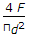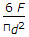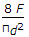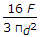# Mechanical Engineering - Machine Design

### Exercise :: Machine Design - Section 7

1.

When a circular beam of diameter d is subjected to a shear force F, the maximum shear stress induced will be

 A.B.C.D.Explanation:

No answer description available for this question. Let us discuss.

2.

In a belt drive, if the pulley diameter is doubled keeping the tension and belt width constant, then it will be necessary to

 A. increase the key length B. increase the key depth C. increase the key width D. decrease the key length

Explanation:

No answer description available for this question. Let us discuss.

3.

Which of the following statement is wrong ?

 A. The solid length of a spring is the product of total number of coils and the diameter of the wire B. The spring index is the ratio of mean diameter of the coil to the diameter of the wire C. The spring stiffness is the load required per unit deflection of the spring D. The pitch of the coil is the axial distance between adjacent coils in the compressed state

Explanation:

No answer description available for this question. Let us discuss.

4.

The draw of cotter should not be more than

 A. 3 mm B. 6 mm C. 8 mm D. 12 mm

Explanation:

No answer description available for this question. Let us discuss.

5.

The bolts of smaller diameter than M16 are not permitted in making fluid tight joints.

 A. Correct B. Incorrect